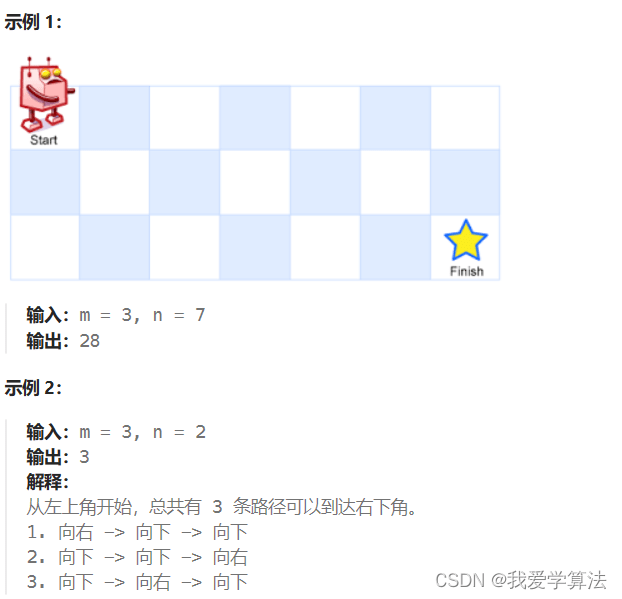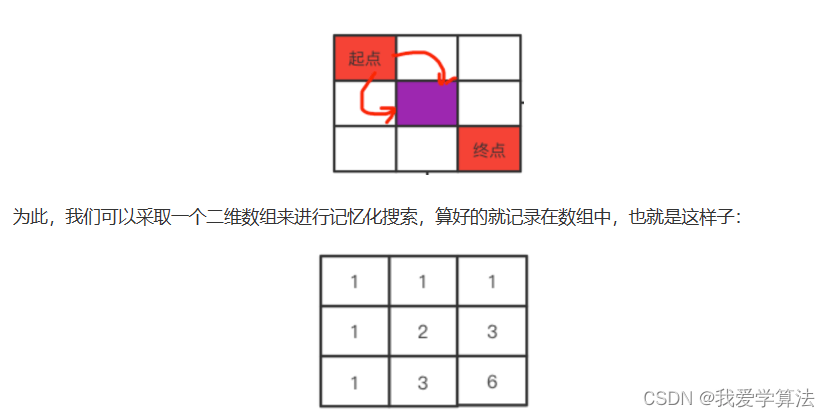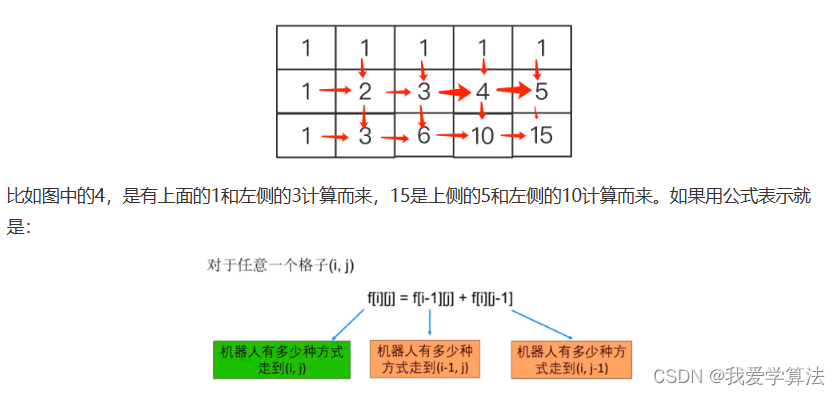# Algorithm Clearance Village | Thorough understanding of dynamic programming

## 1. Fibonacci Sequence

1, 1, 2, 3, 5, 8, 13, ….. f(n) = f(n-1) + f(n-2)

Code implementation

``` public static int count_2 = 0;
public int fibonacci(int n){
if (n <= 2){
count_2 + + ;
return n;
}
int f1 = 1;
int f2 = 2;
int sum = 0;
for (int i = 3; i < n; i + + ) {
count_2 + + ;
sum = f1 + f2;
f1 = f2;
f2 = sum;
}
return sum;
}```

When n=20, the count is 21891 times. When n=30, the result is 2692537, which is close to 2.7 million. Why is it so high? Because there are a lot of loops in it. In the figure below, when n=8, f(6) is Repeat the calculation twice, and many nodes will be repeatedly calculated.How to optimize it and reduce repeated calculations is also what needs to be considered in the following derivation of dynamic programming. The calculation results can be saved in the array, and the value of f(n) is saved in the array. f(n)=arr[n], a certain The position has been calculated and can be taken out directly when needed next time without any need for recursion.

``` public static int[] arr = new int;
public static int count_3 = 0;

public static void main(String[] args) {
Arrays.fill(arr,-1);
arr = 1;
System.out.println(fibonacci(31));
}
static int fibonacci(int n){
if (n == 2 || n == 1){
count_3 + + ;
arr[n] = n;
return n;
}
if (arr[n] != -1){
count_3 + + ;
return arr[n];
}else {
count_3 + + ;
arr[n] = fibonacci(n - 1) + fibonacci(n - 2);
return arr[n];
}
}```

## 2 Path Serial Cannon

We refer to dynamic programming in this article as DP. We analyze dynamic programming with path-related issues. The path problem is easy to draw and understand. You can understand DP step by step.

### 2.1 The first shot: the total number of statistical paths for basic questions

LeetCode62 A robot is located in the upper left corner of a `m x n` grid (the starting point is marked “Start” in the image below).

The robot can only move one step down or to the right at a time. The robot attempts to reach the lower right corner of the grid (labeled “Finish” in the image below).

How many different paths are there in total?For the first shot, we study how to solve this problem through recursion. As shown in the figure below, starting from the starting point is either to the right or downward. Each of these will cause the remaining interval to be reduced by one column or one row, forming two different In the process of intervals, each interval continues the above operation starting from the red dot, so this is a recursive process.Find the pattern in the picture. From the starting point to the end point, the target can only go to the right or downward.

1. Take a step to the right. The gray area below the starting point will no longer be visited. There is a 3X1 matrix left behind. You can only go straight down. There is only one path.

2. Go one step down, and the right side of the starting point can no longer be accessed, leaving a 2X2 matrix and two paths left.

This is a 3X2 matrix, with a total of 3 paths, a 2X2 matrix and the sum of 3X1 matrix paths.Similarly, we derive a 3X3 matrix, which is the sum of a 3X2 matrix and 2X3 paths,

Therefore, for an mxn matrix, the method for finding the path is search(m,n)=search(m-1,n) + search(m,n-1);

``` public int uniquePath(int m,int n){
return search(m,n);
}

private int search(int m, int n) {
if (m == 1 || n == 1){
return 1;
}
return search(m-1,n) + search(m,n-1);
}```

For a 3X3 matrix, we can use a binary tree to representWe can find that, as we mentioned at the beginning of the article, there are repeated calculations, 1 and 1 are calculated twice, so how to optimize

### 2.2 The second shot: using two-dimensional arrays to optimize recursion

We know that there is repeated calculation above. {1,1} appears twice. At the position {1,1}, whether it comes from {0,1} or {1,0}, there will be two moves. We You can use two-dimensional array memory search to eliminate the need to traverse twice.Each grid represents several ways from the starting point to the current position. In this way, when calculating the path, we can first check whether there is a record in the two-dimensional array, and if so, read it directly without further calculation, so that we can Avoid a lot of repeated calculations, this is memorized search.

From the above analysis we get two rules:

1. The first row and column are both 1.

2. The values of other grids are the sum of the grids to the left and above them, and are suitable for mXn grids.``` public int uniquePath(int m, int n){
int[][] f = new int[m][n];
f = 1;
for (int i = 0; i < m; i + + ) {
for (int j = 0; j < n; j + + ) {
if (i > 0 & amp; & amp; j > 0){
f[i][j] = f[i -1][j] + f[i][j -1];
}else if (i > 0){
f[i][j] = f[i -1][j];
} else if (j > 0) {
f[i][j] = f[i][j -1];
}
}
}
return f[m -1][n -1];
}```

### 2.3 The third shot: rolling array: using one dimension instead of two-dimensional array

In the third shot, we optimize this problem by rolling the array. The cache space above uses a two-dimensional array, which takes up a lot of space. If we can further optimize it, let’s look at the two-dimensional array above to find out the rules.It is found that except for the first row and the first column, which are both 1, each position is the sum of the grids to its left and above, which can be solved with a one-dimensional array of size n:Splicing several one-dimensional arrays together is exactly the same as the two-dimensional array above. The strategy for repeatedly updating the array is to roll the array. The calculation formula is: dp[j] = dp[j] + dp[j-1]

``` public int uniquePaths(int m, int n){
int[] dp = new int[n];
//Initialize the initial element of the array to 1
Arrays.fill(dp,1);
for (int i = 0; i < m; i + + ) {
for (int j = 0; j < n; j + + ) {
//The dp[j] on the left side of the equation is after the last calculation, plus the dp[j-1] on the left side is the current result
dp[j] = dp[j] + dp[j - 1];
}
}
return dp[n -1];
}```

This question includes many aspects of DP (dynamic programming), such as repeated subproblems, memorized search, rolling arrays, etc. This is the simplest dynamic programming, but our planning here is dp[j] = dp[j] + dp[j -1]; No need to perform complex comparisons and calculations.

## 3. Understand dynamic programming

DP generally allows us to find the best value, such as the longest common subsequence. The most important thing is that the sub-problems of the DP problem are not independent of each other. If the recursion is directly decomposed, it will lead to an exponential increase in repeated calculations. The warm-up questions at the beginning, and DP The greatest value is to eliminate redundancy and speed up calculations.

What problem does dynamic programming solve: A finds how many moves there are, and B outputs all moves

Dynamic programming has high computational efficiency, but it cannot find a path that meets the requirements.

The most important thing to distinguish between dynamic programming and recursion is: Dynamic programming only cares about what the current result is, and does not care how it came about. Therefore, dynamic programming cannot obtain a complete path. This is different from backtracking, which can obtain an even path. All complete paths that meet the requirements.

The basic idea of DP is to decompose the problem to be solved into several sub-problems, first find the sub-problems, and then get the solution to the original problem from these sub-problems. Since you want to find the best value, what you must do is to exhaustively Look for all possibilities, and then choose the “most” one. This is why a lot of judgment logic in DP code is covered with min() or max().

Since it is exhaustive, why is there still the concept of DP? This is because there are a lot of repeated calculations in the exhaustive process, which is inefficient, so we need to use methods such as memorized search to eliminate unnecessary calculations. The so-called memorized search is to store the calculated results in the array first, and then Just read it directly and don’t need to repeat calculations.

Since memorization can solve the problem, why is DP so difficult? Because the DP problem must have an “optimal substructure” so that accurate results can be obtained during memorization. As for when the optimal substructure is, there are specific questions later. ,

With the optimal substructure, you need to have the correct “state transition equation” to correctly enumerate, that is, the recursive relationship. Most recursion can be realized through arrays, so the code structure is generally a for loop like this, This is the basic template of DP:

//Initialize base case, that is, the first few scenarios, there are several enumerations
dp[…] = base case
//Perform state transfer
for state 1 all values of state 1
for state 2 in all values of state 2
dp[State 1][State 2] = Find the maximum value Max(Select 1, Select 2,…)

There are three basic types of dynamic programming questions:

1. Counting related, for example, how many ways to get to the lower right corner, how many ways to select K numbers, what is the problem, not caring about the path.

2. Find the maximum and minimum values, most and least, etc., such as the maximum sum of numbers, the longest ascending subsequence, the longest common subsequence, the longest palindrome sequence, etc.

3. Seeking existence, such as the game of picking stones, whether the first player will definitely win, whether k numbers can be selected to make what and so on, etc.

No matter what kind of problem-solving template it is, it is similar.

• The first step: Determine the status and sub-problems, that is, enumerate all possibilities at a certain position. For DP, the last step of most problem analysis is easier, obtaining the recursion relationship and converting the problem into sub-problems.
• Step 2: Determine the state transition equation, that is, what content is to be stored in the array. In many cases, after the state is determined, the state transition equation is also determined. The first two steps can also be regarded as the first step.
• Step 3: Determine the initial and boundary conditions, be careful and as thorough as possible
• Step 4: Calculate in order from small to large: f, f, f

From beginning to end, we have to install an array in our brain (It may be one-dimensional or two-dimensional), and it depends on the meaning of each element of this array (that is, state< /strong>), it depends on who calculates each array (state transition equation), and then fills the arrays from small to large (calculates from small to large, to achieve memory search ), finally looking at that position is the result we want.